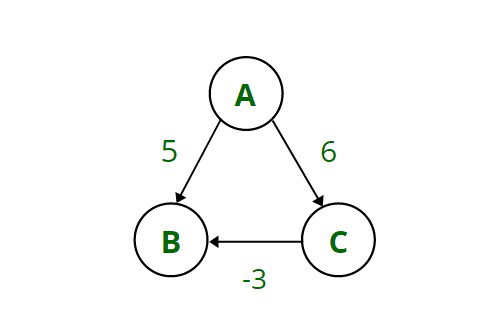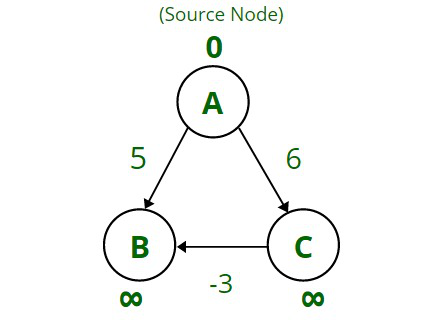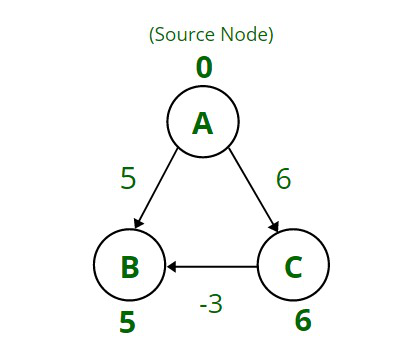# Why does Dijkstra’s Algorithm fail on negative weights?

• Difficulty Level : Medium
• Last Updated : 28 Nov, 2021

Dijkstra’s Algorithm: It is a graph searching algorithm that uses a Greedy Approach to find the shortest path from the source node to all other remaining nodes. It solves the single-source shortest path problem for a weighted graph. This algorithm keeps track of the weights of the edges for finding the path that minimizes the total distance.

Time Complexity: O(V + E*log(V)), when priority queue is used (where V are the nodes and E are the edges)

Limitations of Dijkstra’s Algorithm: For this algorithm to function properly:

• The graph should be weighted and directed.
• The weights should be non-negative.

• It has a linear time complexity so it can be easily used for large problems.
• It is useful in finding the shortest distance, so it is also used in google maps and calculating traffic.

• It is unable to handle negative weights.
• It follows a kind of blind approach so there is a wastage of time.

Why does Dijkstra’s Algorithm fail on negative weights?

Let’s take a simple example for a better understanding of why Dijkstra’s Algorithm fails for negative weights.Consider cyclic directed graph with nodes A, B, and C which is connected by edges having weights that represent the cost to use this edge. The following are the weights as mentioned in the above diagram:

A –>B = 5, A –>C = 6, C –>B = -3. Here one weight C -> B is negative.

• Consider node A as the source node and the task is to find the shortest distance from source node A to all the other nodes present in the graph i.e., nodes B and C.• So, first mark the distance as 0, at node A (as the distance from A to A is 0), and then mark this node as visited meaning that it has been included in the shortest path.
• Since in the beginning, the distance of the source node to all other nodes is not known so initialize it as infinity. Update this distance if  any distance shorter than infinity is found (which is basically the greedy approach)• Then, update the distance from source node A to B with the weight of the edge that connects it with A which is 5 (because 5 < infinity).
In a similar way, also update the distance from A to C which was previously infinity to 6 (as 6 < infinity).
• Now check for the shortest distance from the source node A and as 5 is the least distance from A to B, so mark node B as ‘visited‘.
Similarly, the next shortest is 6 so mark node C also as visited. At this point, all three nodes of the graph are visited.
• Now the most important step arises here, as it can be seen that by following this algorithm, the shortest distance from A –> B is 5 but if traveled the distance via node C that is the path A –> C –> B the distance will be as 3 (as A–>C = 6 and C–>B = -3 ), so 6 + (-3) = 3. As 3 is less than 5, but Dijkstra’s algorithm gives the incorrect answer as 5, which is not the shortest distance. Therefore Dijkstra’s Algorithm fails for negative cases.

Conclusion:

• Since Dijkstra follows a Greedy Approach, once a node is marked as visited it cannot be reconsidered even if there is another path with less cost or distance. This issue arises only if there exists a negative weight or edge in the graph.
• So this algorithm fails to find the minimum distance in case of negative weights, so as an alternative Bellman-Ford algorithm is used to find the shortest distance in case of negative weights, as it stops the loop when it encounters a negative cycle.
My Personal Notes arrow_drop_up